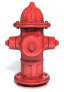Name:    1.MD1.1

Multiple Choice
Identify the choice that best completes the statement or answers the question.

1.

Which object is about 5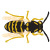long?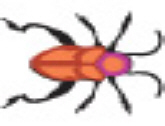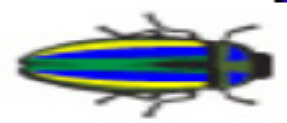a.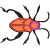b.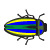2.

Which of the following is about 3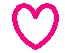in height?a.b.3.

Which is about the same size as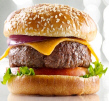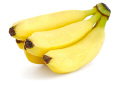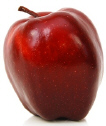a.b.4.

Which one is about 4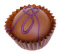in length?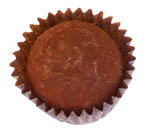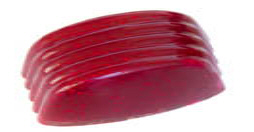a.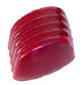b.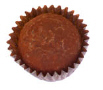5.

Which one is about 2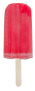in height?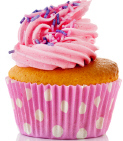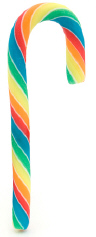a.b.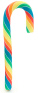6.

Which one is about 3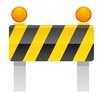in length?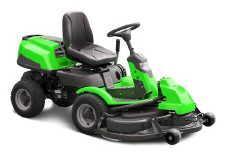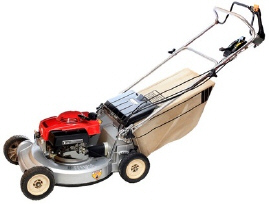a.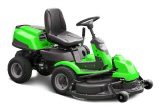b.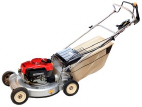7.

Which one is about 2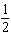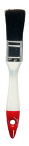in length?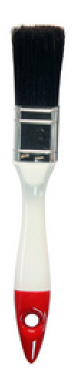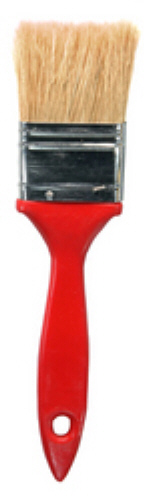a.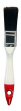b.8.

Which of these is the same height as 2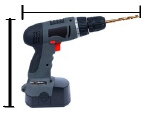in length?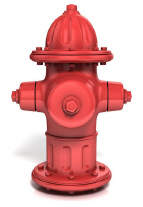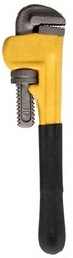a.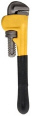b.